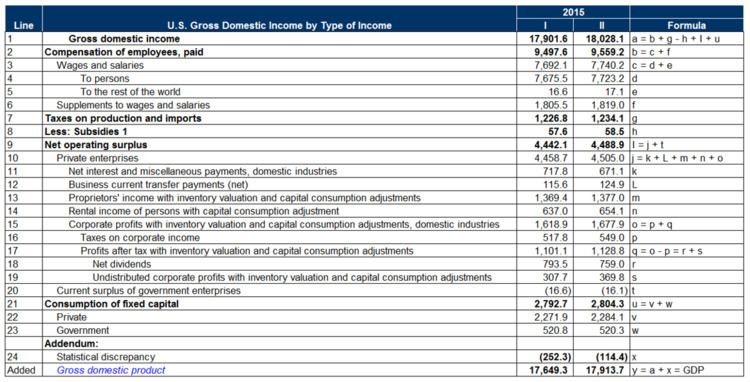# Gross domestic income

Updated onThe Gross Domestic Income (GDI) is the total income received by all sectors of an economy within a state. It includes the sum of all wages, profits, and taxes, minus subsidies. Since all income is derived from production (including the production of services), the gross domestic income of a country should exactly equal its gross domestic product (GDP). The GDP is a very commonly cited statistic measuring the economic activity of countries, and the GDI is quite uncommon.

In the United States, the Bureau of Economic Analysis produces figures for both the GDP and GDI. Although these should be equal, since they are calculated in different ways, in practice, the listed figures are different. This difference is known as the statistical discrepancy.

Topics